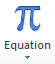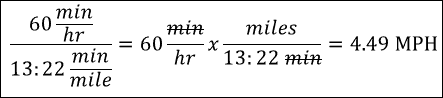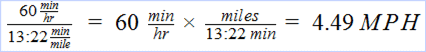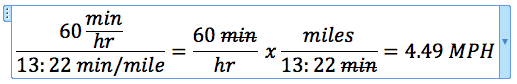Equation Editors in Excel 2010 and Word 2011The Equation Editor is new in Excel 2010 and designed to create a mathematical equation as a graphical object. I didn’t find it in Excel 2011 for Mac, but ran across an article showing they put it inside Word 2011. How very strange.

Here I’ll review the Excel 2010 version of Equation Editor first, then briefly compare Mathematical Equations in Google Docs, and come back to the Word 2011 version of Equation Editor.

Excel 2010 Equation Editor

The Equation Editor in Excel 2010 is difficult to navigate at first. The second time I tried creating an equation it got slightly easier, and the third time with the same equation (shown below) I seemed to almost get the hang of it.The trick I learned was to just type the equation in and let the Editor do its thing in rearranging what you’re typing. On the Equation Tools Ribbon are two helpful selections: Professional and Linear (shown below). Playing around with these helped me understand how the Editor can manipulate simple equations.

Having said that, advanced equations will take some time to figure out, but this editor should handle most anything you can throw at it.

Entering an Equation

To enter an equation with the Equation Editor go to the Insert tab on the Ribbon, and click Equation. This gives you a text box with the words “Type equation here.” You’ll also notice two additional menu tabs on the Ribbon: Equation Tools, which becomes active, and Drawing Tools.

There are varied Symbols readily available for insertion on the Equation Tools Ribbon tab (shown above). And several drop-down menu items that expand to show even more symbols for things like: Fraction, Script, Radical, Integral, Large Operator, Bracket, Function, Accent, Limit and Log, Operator, and Matrix. (Shown below)

Clicking the Equation drop-down arrow will reveal several predefined equations that can be inserted as starting point for your equation. This how I started my first equation, which did me no favors. I fared much better just typing in the formula and letting the Equation Editor move things around.

One Annoyance

One of the problems with the Equation Editor is that if you stray to far outside the equation, yet still inside the text box, the Equation Tools tab disappears and you’re stuck with the Drawing Tools tab only. Maddening!

I looked at the new Mathematical Equations in Google Docs and it seemed easier to learn, but is not nearly as powerful as the Equation Editor. For example, the equation I created above was done in a Google Docs document with Mathematical Equations. I wasn’t able to do a strikethrough for the min text, like this: min.And there wasn’t a good way to export this object out of Google Docs.

Equation Editor in Word 2011 for Mac

The Equation Editor in Word 2011 for Mac looks to be identical to the one in Excel 2010. You start an equation from the Document Elements tab on the Ribbon, by clicking Equation or the drop-down menu arrow and selecting a built-in equation.

The Equation Tools tab immediately becomes visible and active after starting an equation. I created the same formula with relative ease.I kind of like the feel of this Word 2011 version better than what’s in Excel 2010. Go figure.